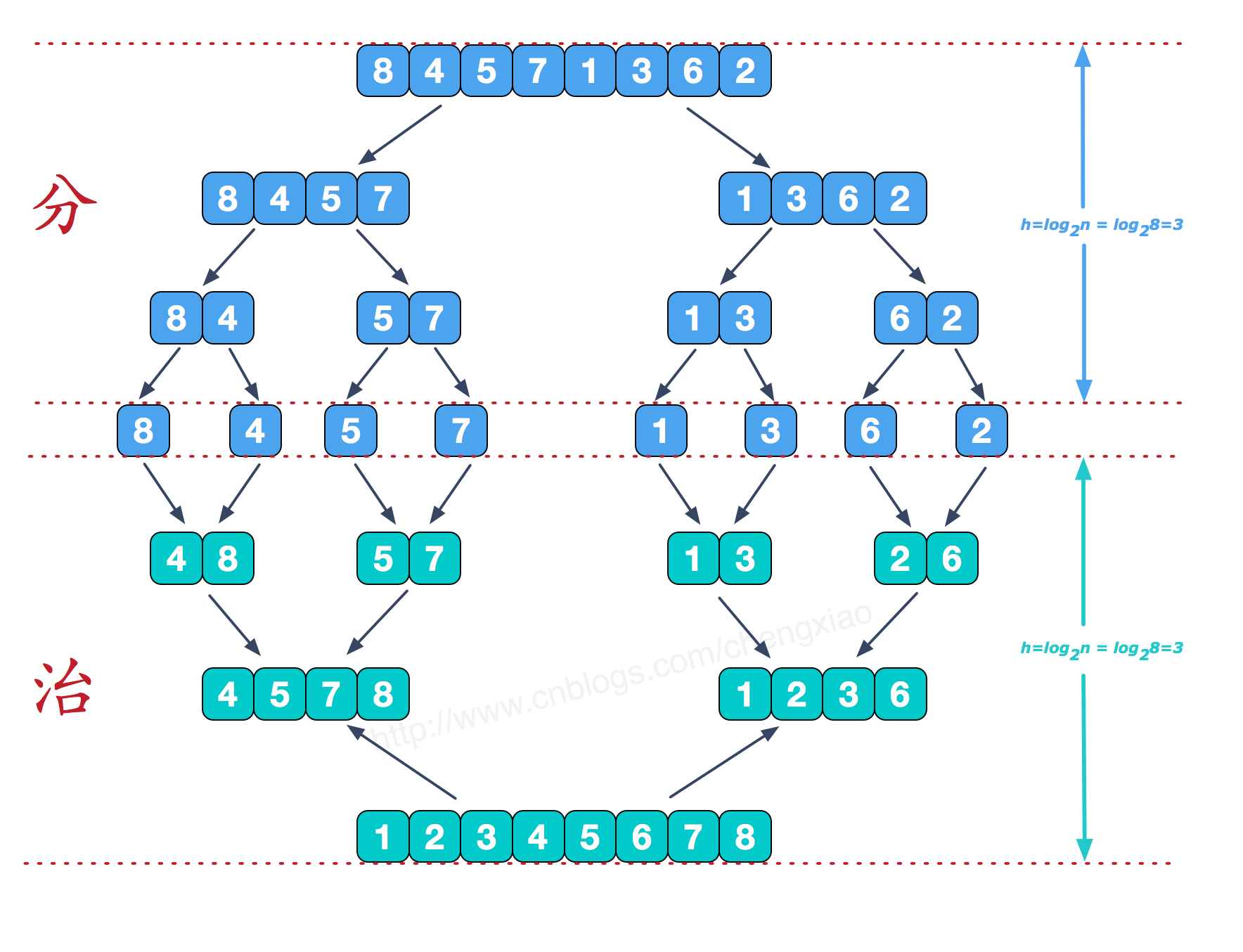归并排序：

归并排序（MERGE-SORT）是利用归并的思想实现的排序方法，该算法采用经典的分治（divide-and-conquer）策略（分治法将问题(divide)成一些小的问题然后递归求解，而治(conquer)的阶段则将分的阶段得到的各答案"修补"在一起，即分而治之)。

-----------------------------------------------------------------------------------------------------ps:由于拆分到最小单位，成为1个数组只有1个 数字 的时候，合并，两个数字的合并。

-------------------------------------------------------------------------------------

递归核心理解：

首先贴出来核心代码：

public static void main(String[] args) {
//准备两个排好顺序的数组
int[] arr1={1,3,7,9,55,999};
int[] arr2={2,5,11,33,44,100,1000};
//放排序好的数组
int[] arr3=new int[arr1.length+arr2.length];
//控制arr1
int i=0;
//控制arr2
int j=0;
//控制arr3
int n=0;
while(i<arr1.length && j<arr2.length){
if(arr1[i]<arr2[j]){
//如果arr1 数组当前的数字小于arr2
//就把arr1的当前数字 放入arr3中
arr3[n++]=arr1[i++];
}else{
//如果arr2 数组当前的数字小于arr1
//就把arr2的当前数字 放入arr3中
arr3[n++]=arr2[j++];
}
}
//由于可能数组长度不同，或者 某个数组还未将其余的放入arr3中
//如果i没有循环到 结尾 ，就 将其余的放入 arr3 中 ，
//无需担心排序，因为之前就已经有序。
while(i<arr1.length){
arr3[n++]=arr1[i++];
}
while(j<arr2.length){
arr3[n++]=arr2[j++];
}
System.out.println(Arrays.toString(arr3));
}

----------------------------------------------------------------------------------------

归并排序代码

import java.util.Arrays;
import java.util.Random;

public class guibing {
public static void main(String[] args) {
int[] arr = new int;
for (int i = 0; i < arr.length; i++) {
arr[i] = new Random().nextInt(100);
}
sort(arr);
System.out.println(Arrays.toString(arr));
}

private static void sort(int[] arr) {
int[] temp = new int[arr.length];
sort(arr, 0, arr.length - 1, temp);
}

private static void sort(int[] arr, int left, int right, int[] temp) {
//当数组拆分成1个的时候，就是 递归拆分结束。
if (left < right) {
// 将数组从中间分开 成为2个数组
int mid = (left + right) / 2;
// 递归拆分左边
sort(arr, left, mid, temp);
// 递归拆分右边边
sort(arr, mid + 1, right, temp);
// 合并两个数组
hebing(arr, left, mid, right, temp);
}

}

private static void hebing(int[] arr, int left, int mid, int right, int[] temp) {
int i = left;
int j = mid + 1;
int n = 0;
while (i <= mid && j <= right) {
// 归并排序的核心
if (arr[i] < arr[j]) {
temp[n++] = arr[i++];
} else {
temp[n++] = arr[j++];
}
}
// 如果某组还有剩余，将剩余的放入
while (i <= mid) {
temp[n++] = arr[i++];
}
while (j <= right) {
temp[n++] = arr[j++];
}
// 将排序好的数组放回去
n = 0;
while (left <= right) {
arr[left++] = temp[n++];
}
}
}

--------------------------------------------------------------------

http://www.92to.com/bangong/2016/11-28/13707795.html 推荐只看图。

http://www.cnblogs.com/chengxiao/p/6194356.html  推荐全看。

----------------------------------------------------------------------暂无文章

TiDB
19分钟前
5
0
jquery qrcode库提示not function

jquery qrcode 这个库能用，但是必须在初始化的时候，官方给的使用方法是 引入qrcode的库文件后，在js中写以下 html <div id="qrcode"></div> js jQuery('#qrcode').qrcode({ render: ......

shikamaru
24分钟前
9
0
MySQL数据库去重的简单方案

FeanLau
26分钟前
9
0

37分钟前
8
0
Eureka客户端续约及服务端过期租约清理源码解析

Java学习录
49分钟前
10
0